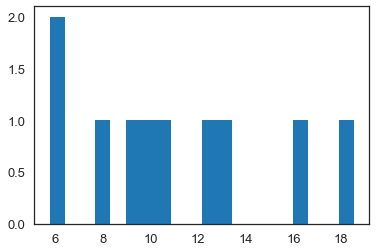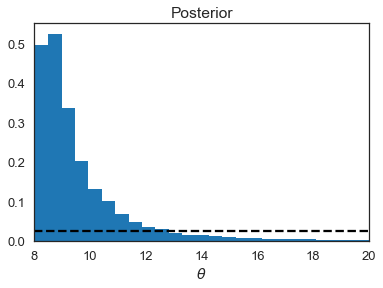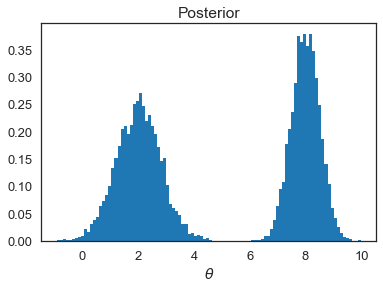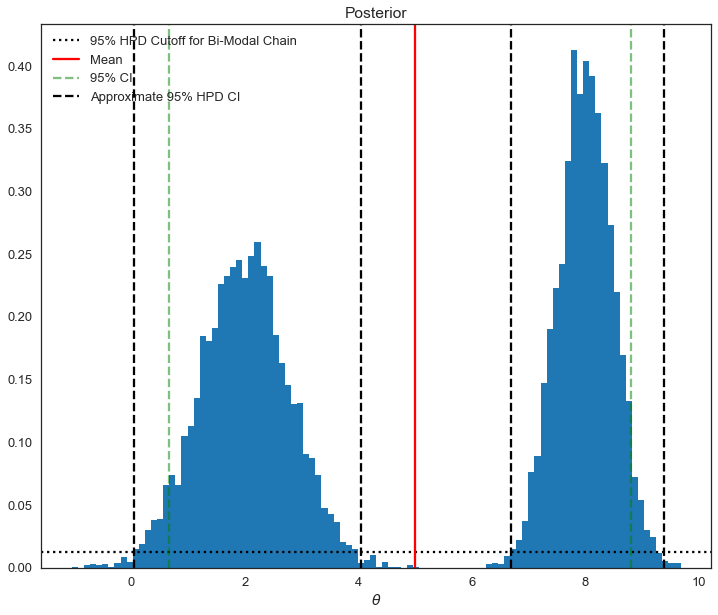# Inference

In :
%matplotlib inline
import numpy as np
from scipy.stats import norm,uniform,lognorm
import matplotlib.pyplot as plt
import seaborn as sbn
import pandas as pd
import pymc3 as pm3
import warnings
warnings.filterwarnings('ignore')

np.random.seed(348348756)

sbn.set_style('white')
sbn.set_context('talk')

/home/robhicks/anaconda/envs/python36/lib/python3.6/site-packages/h5py/__init__.py:36: FutureWarning: Conversion of the second argument of issubdtype from float to np.floating is deprecated. In future, it will be treated as np.float64 == np.dtype(float).type.
from ._conv import register_converters as _register_converters


For having some data to play with, let's resurrect our simple example yet again:

In :
data = norm(10,3).rvs(10)
sigma = 3          # Note this is the std of x
mu_prior = 8
sigma_prior = 1.5  # Note this is our prior on the std of mu

plt.hist(data,bins=20)
plt.show()In :
# a fairly basic mh mcmc random walk sampler:
def sampler(data, samples=4, mu_init=9, sigma= 1, proposal_width=3,
mu_prior_mu=10, mu_prior_sd=1):
mu_current = mu_init
posterior = [mu_current]
for i in range(samples):
# suggest new position
mu_proposal = norm(mu_current, proposal_width).rvs()

# Compute likelihood by multiplying probabilities of each data point
likelihood_current = norm(mu_current, sigma).pdf(data).prod()
likelihood_proposal = norm(mu_proposal, sigma).pdf(data).prod()

# Compute prior probability of current and proposed mu
prior_current = norm(mu_prior_mu, mu_prior_sd).pdf(mu_current)
prior_proposal = norm(mu_prior_mu, mu_prior_sd).pdf(mu_proposal)

p_current = likelihood_current * prior_current
p_proposal = likelihood_proposal * prior_proposal

# Accept proposal?
c = np.min((1,p_proposal / p_current))

accept = np.random.rand() <= c

if accept:
# Update position
mu_current = mu_proposal

posterior.append(mu_current)

return np.array(posterior)

In :
chain = sampler(data,samples=5000,mu_init=9,sigma=sigma,proposal_width=3,
mu_prior_mu=mu_prior,mu_prior_sd = sigma_prior)


### Using our Chain for Inference¶

In what follows, I assume we have already assessed chain convergence and are satisfied.

In the code below, I ask a number of questions (you might call this inference) using our sampled posterior values, starting with ones most similar to frequentist statistics:

In :
# exclude first 100 iterations for burn-in
chain_s = chain[100:]

In :
print("The mean is: ", chain_s.mean())
print("The standard deviation is: ", chain_s.std())
print("The median is: ", np.percentile(chain_s,50))
print("The 95% Credible Interval is: ",np.percentile(chain_s,[2.5,97.5]))
print("The probability that the mean is greater than 10: ", \
chain_s[chain_s>10].shape/chain_s.shape)

The mean is:  10.180621628544616
The standard deviation is:  0.8093876372087345
The median is:  10.196002242838691
The 95% Credible Interval is:  [ 8.51523653 11.77003799]
The probability that the mean is greater than 10:  0.6015098959396041


While the last question isn't one we typically ask in a frequentist setting, we could by imposing normality from our model estimates and calculating areas under the pdf. Alternatively, we could perform a bootstrapping excercise as we did earlier in the class. The posterior pdf pictured above does not assume normality of our parameters and in that way is similar to the bootstrap approach for inference.

The Credible Interval above can be interpreted as

There is a 95% chance that $\theta$ is in the interval [8.84,9.95]

Note: the numbers in brackets will change depending on the credible interval printed above.

##### Classical Hypothesis testing¶

In frequentist statistics, we often perform test as follows:

$$H_0 : \theta = 0 \\ H_1 : \theta \ne 0$$

This isn't very easy to do in a Bayesian Context. We can see that no values of our chain is exactly equal to 0 and this would likely be the case even if our mean for this problem was centered on zero.

To make a hypothesis test more relevant for our problem, recast to $$H_0 : \theta = 10 \\ H_1 : \theta \ne 10$$

To see this point, note that there are no values of $\theta$ in our chain exactly equal to 10:

In :
print("Number of cases where theta=10: ", chain_s[chain_s==10].shape)

Number of cases where theta=10:  0


So does this mean we have 100% evidence that theta isn't 10? A tough question to answer. A better question might be to recast this question into an interval:

$$H_0 : \theta \in 10 \pm .1 \\ H_1 : \theta \notin 10 \pm .1$$
In :
print("Probability of H_0: ",chain_s[(chain_s<=10.1) & (chain_s>=9.9)].shape / chain_s.shape)

Probability of H_0:  0.09610283615588655


Another type of question we might answer are hypothesis tests over intervals.

A more common question answered in a Bayesian setting is: $$H_0 : \theta < 10 \\ H_1 : \theta \text{ not } < 10$$

In :
print("Probability of H_0: ",chain_s[chain_s<10].shape / chain_s.shape)

Probability of H_0:  0.3984901040603958


So Bayesians like to attach probabilities to statements. Additionally note that due to our very small sample size, the prior is definitely affecting our inference here.

###### Highest Posterior Density (HPD) Intervals¶

For skewed or multimodal posteriors, credible intervals may not be as enlightening as they could be. Suppose we have a MH markov chain and our posterior pdf is plotted below. The HPD interval, sets a minimum probability threshold for parameters (e.g. posterior value must be greater than .025) and examines the interval of values of $\theta$ that are

In :
skewed_chain = lognorm(1,8).rvs(10000)
ax = plt.hist(skewed_chain,bins=100,normed=True)
ax = plt.axhline(.025, c='k', linestyle='--')
plt.xlabel('$\\theta$')
plt.xlim((8,20))
plt.title('Posterior')
plt.show()In :
print("The mean is: ", skewed_chain.mean())
print( "The standard deviation is: ", skewed_chain.std())
print("The median is: ", np.percentile(skewed_chain,50))
print("The 95% Credible Interval is: ",np.percentile(skewed_chain,[2.5,97.5]))

The mean is:  9.643799028459755
The standard deviation is:  2.1150266705678
The median is:  9.011681077018235
The 95% Credible Interval is:  [ 8.15066093 15.03739996]

In :
print("The highest posterior density interval is: ")
print(pm3.hpd(skewed_chain))
print("Note: Min Chain Value is: ",skewed_chain.min())

The highest posterior density interval is:
[ 8.04731833 13.1198713 ]
Note: Min Chain Value is:  8.025551305915142

In :
# suppose our chain was this
bimodal_chain = np.append(norm(2,.8).rvs(5000),norm(8,.5).rvs(5000))
plt.hist(bimodal_chain,bins=100,normed=True)
plt.xlabel('$\\theta$')
plt.title('Posterior')
plt.show()In :
print("The highest posterior density interval is: ")
print(pm3.hpd(bimodal_chain))

The highest posterior density interval is:
[0.75956428 8.85741972]


The pymc hpd function is not built for multi-modal chains.

The following shows what it should be:

In :
plt.figure(figsize=(12,10))
bimodal_chain = np.append(norm(2,.8).rvs(5000),norm(8,.5).rvs(5000))
plt.hist(bimodal_chain,bins=100,normed=True)
plt.xlabel('$\\theta$')
plt.title('Posterior')
plt.axhline(.025/2,c='k',linestyle=':',label="95% HPD Cutoff for Bi-Modal Chain")
plt.axvline(bimodal_chain.mean(),c='r',label="Mean")
plt.axvline(np.percentile(bimodal_chain,2.5),c='g',linestyle='--',alpha=.5,label="95% CI")
plt.axvline(np.percentile(bimodal_chain,97.5),c='g',linestyle='--',alpha=.5)

plt.axvline(.05,c='k',linestyle='--',label="Approximate 95% HPD CI")
plt.axvline(4.05,c='k',linestyle='--')
plt.axvline(6.7,c='k',linestyle='--')
plt.axvline(9.39,c='k',linestyle='--')

plt.legend(loc='upper left')
plt.show()#### The Chain and MAP¶

How do the moments of our chain (e.g. mean and median) relate to MAP? To answer that question, we need to solve for MAP given the data:

In :
def log_posterior(mu,data,sigma,mu_mu_prior,mu_sigma_prior):
ln_prior = norm(mu_mu_prior,mu_sigma_prior).logpdf(mu)
ln_like = norm(mu,sigma).logpdf(data).sum()
return -1*(ln_like + ln_prior)

In :
startvals = 9
from scipy.optimize import minimize
res = minimize(log_posterior,startvals,
args=(data,sigma,mu_prior,sigma_prior),options={'disp': True},
method='L-BFGS-B')

In :
print("The MAP is: ", res.x)
print("The mean is: ", chain[100:].mean())
print("The median is: ", np.percentile(chain[100:],50))

The MAP is:  10.16031563320772
The mean is:  10.180621628544616
The median is:  10.196002242838691


For well behaved symmetric posteriors, the mean of the posterior will usually be very close to MAP, but this doesn't necessarily have to be the case. Note that in the preceding figure (the bimodal posterior), MAP is approximately 8 whereas the mean is approximately 5.